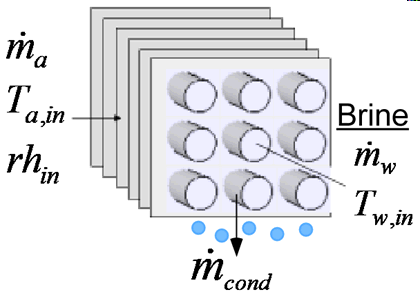Contents - index

CoolingCoil2_CLCoolingCoil2_CL models the performance of a heat exchanger designed to cool humid air in which the air is cooled by heat exchange with a brine solution.  The brine is selected from any of the brine fluids built into EES.    Other than using brine instead of pure water, this model is the same as that used for CoolingCoil1_CL.

Inputs:

m_dot_a:  mass flow rate of dry air (kg/s, lbm/hr)

T_a_in:  inlet air temperature (C, F, K, R)

rh_in:  inlet air relative humidity

P:  atmospheric pressure (Pa, kPa, bar, MPa, atm, psia)

F\$: name of the brine mixture, e.g., EG or PG

C: concentration of the brine solution (%)

m_dot_b: mass flow rate of bine solution (kg/s, lbm/hr)

T_b_in:  inlet brine temperature  (C, F, K, R)

U_a:  heat transfer coefficient on air side of coil (W/m^2-K, Btu/hr-ft^2-R)

A_a:  heat transfer area on air side of coil (m^2, ft^2)

U_b:  heat transfer coefficient on brine side of coil (W/m^2-K, Btu/hr-ft^2-R)

A_b:  heat transfer area on brine side of coil (m^2, ft^2)

Outputs:

Q_dot:  heat transfer rate (W, kW, Btu/hr)

T_a_out:  outlet air temperature  (C, F, K, R)

T_b_out:  outlet brine solution temperature  (C, F, K, R)

m_dot_cond: condensate removal rate (kg/s, lbm/hr)

Example:

\$UnitSystem Eng F psia mass

P = 14.7 [psia] "Pressure"

T_a_in = 80 [F] "Temperature"

T_wb_in = 64 [F] "Temperature"

rh_in=relhum(AirH2O,T=T_a_in,B=T_wb_in,P=P) "relative humidity"

m_dot_a = 21000 [lbm/hr] "Flow rate"

"Brine side variables"

T_b_in =  42 [F] "Temperature"

m_dot_b= 30000 [lbm/hr] "Flow rate"

F\$='EG'

C=30 [%]

"Coil Parameters  The symbol U_a is used for the overall surface effectiveness-heat transfer coefficient product"

U_a = 50 [Btu/hr-F-ft^2] "Unit conductance"

U_b = 1000  [Btu/hr-F-ft^2] "Unit conductance"

A_a = 360 [ft^2] "Area"

A_b = 18 [ft^2] "Area"

Call coolingcoil2_cl(m_dot_a, T_a_in, rh_in, P, F\$, C, m_dot_b,  T_b_in, U_a, A_a, U_b, A_b:Q_dot, T_a_out, T_b_out, m_dot_cond)

{Solution:

m_dot_cond=14.61 [lb_m/hr]

Q_dot=162404 [Btu/hr]

T_a_out=52.45 [F]

T_b_out=48.17 [F]

}